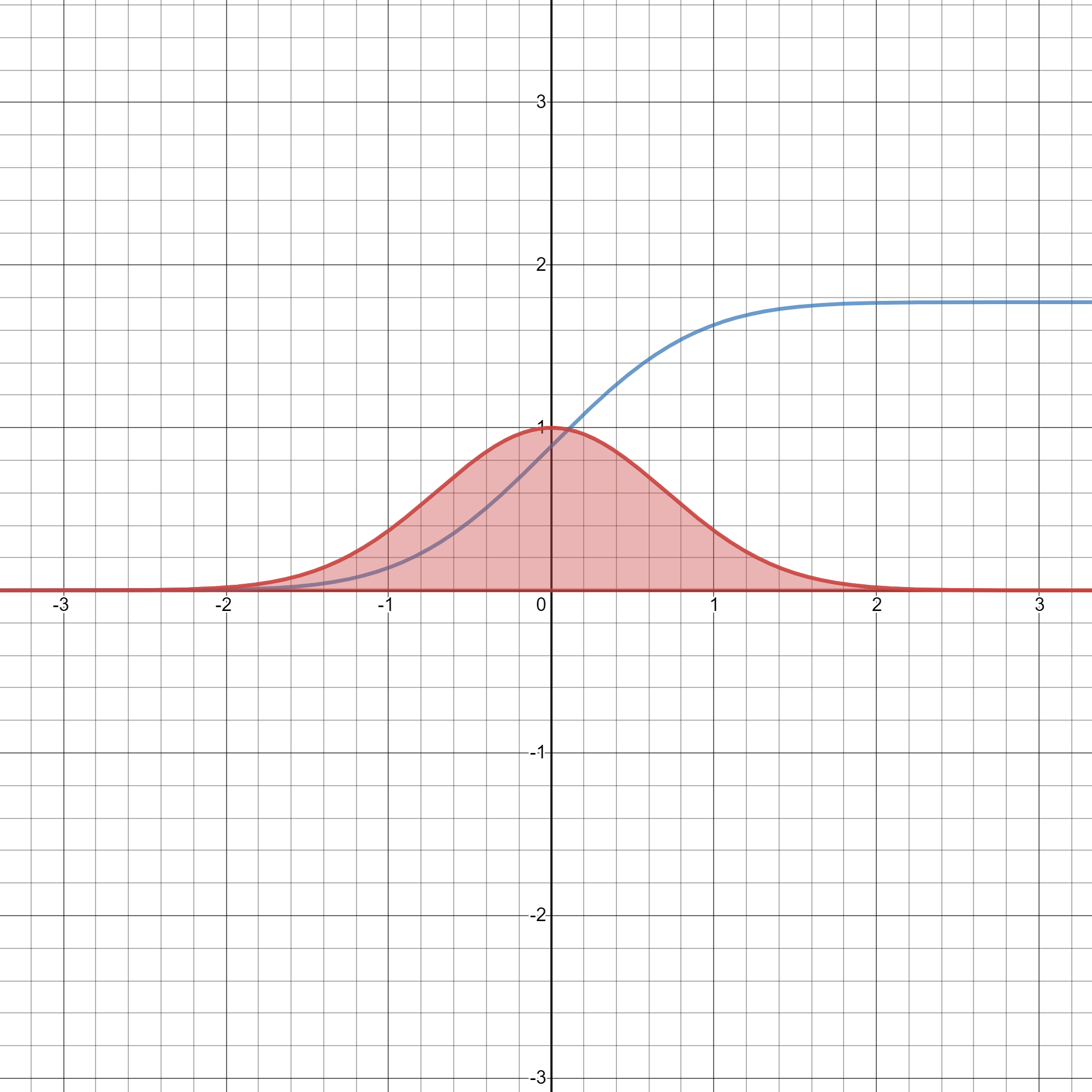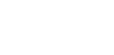# Category: Integration techniques## Gaussian integral using Feynman’s technique

In my last post we evaluated the following definite integral This is the formula we got: and this is the integral we want to evaluate: which is equivalent to because… Read more Gaussian integral using Feynman’s technique## Integral of e^-x^t using Feynman’s technique

Wouldn’t it be nice to generalize the Gaussian integral to any exponent of –x? This is the famous integral: Since this is an even function, it can be written as… Read more Integral of e^-x^t using Feynman’s technique## Integral of sin(x)/x from 0 to infinity | A satisfying solution

Today we have a tough integral: not only is this a special integral (the sine integral Si(x)), but it also goes from 0 to infinity! Because of the first characteristic,… Read more Integral of sin(x)/x from 0 to infinity | A satisfying solution

## Integration techniques | Trigonometric substitution

When none of the techniques you’ve learnt so far (u-substitution 1, 2,3,4 and integration by parts seem to work, you might consider trigonometric substitution. Basically, if you have a function that reminds you of a trigonometric identity, you let that function be equal to the result of the identity, for example cos²x from 1-sin²x, and then differentiate. To make things more clear I’m going to use this example: U substitution and integration by parts are not going to be very helpful. What we are gonna do instead is let x=sin(theta)… Read more Integration techniques | Trigonometric substitution

## Integration techniques | U substitution – part 2

U-substitution is the most common technique used in integration. It can happen, however, that it doesn’t work out, no matter what you try to substitute. You may think of using another technique, like integration by parts, but it’s not always necessary. You realise u-sub is not working when you still see one or more x’s. For example: As you can see, we have an x that we don’t want. At this point you may think about integration by parts, and yes, that’s actually the way to go. The result is… Read more Integration techniques | U substitution – part 2## Integration techniques | Integration by parts

U-substitution is a very useful technique for integration. You can apply it in every case, but it doesn’t always make things easier. But here comes this new technique in help!… Read more Integration techniques | Integration by parts## Integration techniques | U substitution

Ok, we now have the basis of integration! As I said in the previous post, I am now going to cover the techniques of integration. I will make a post… Read more Integration techniques | U substitution

{{#pages}} {{/pages}}
%%footer%%